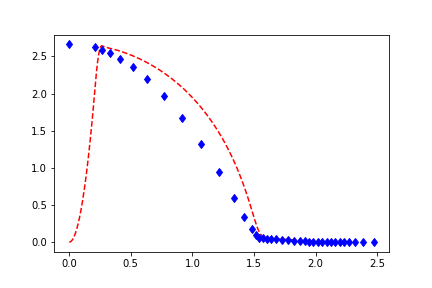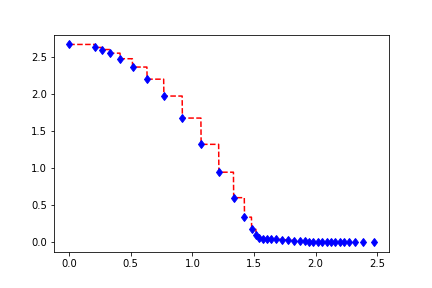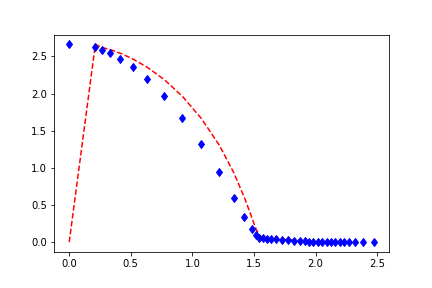# CoefficientFunction from interpolation list

Hello Everyone,

I want to define a CoefficientFunction from another CoefficientFunction using an interpolation list for values.

Here is a short example:

[code]import numpy as np
from ngsolve import *

B_list = [0.0, 0.211862, 0.265665, 0.332377]
mu_list = [2.66233640, 2.62172474, 2.59376841, 2.54489696]
B_cf = x[/code]
Here I tried to define the CoefficientFunction as follows:

``mu_cf = CoefficientFunction( [ np.interp(B, B_list, mu_list) for B in B_cf ] )``

But this does not work.

Is there a way of making the CoefficientFunction make use of the np.interp() function or is there another way?

Thanks
Nils

You can use the BSpline coefficient function for this. It gets an order, a list of nodes and a list of values.
Best
Christopher

Thanks! The BSpline CF probably what I was looking for.

The value of that CF however follows the data not very closely.
I’m setting up the CF like this:

```spline = BSpline(n, B_list, mu_list) mu_cf = spline(B_cf)```
I put the output value of that CF for different orders compared to the interpolation data in the attachments. Order 1 is mapping the the data in a acceptable way but since I need to differentiate this cf order 1 is out of question.

Is there a way of making the CF just interpolate the data or something else to make this work?

Best regards
Nils

Data: blue dots
BSpline result: red dashed line
Order n=1you have to duplicate the first control point (value of B_list)
then the n=2 B-spline will be linear interpolation.

Joachim

Thanks!

Now I have a different problem:

I’m using this CF in a nonlinear BilinearForm:

```u,v = fes.TnT() a = BilinearForm(fes) a += SymbolicBFI(1.0/spline(Norm(grad(u))) * grad(u) * grad(v))```
When assembling a linearized matrix of the system the matrix has a lot of nan entries.
This should not happen since the values of spline() are always nonzero positive.

Do you know why there are nan entries in the matrix and how to avoid it?

Best regards
Nils

difficult to say without testing, but maybe you try to differentiate Norm at grad(u) = 0 ?

``````def RegNorm(func):